## Archive for the 'Engineering' Category

08
Nov
07

### Joukowski Airfoil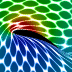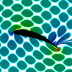These animations were created using a conformal mapping technique called the Joukowski Transformation. A Joukowski airfoil can be thought of as a modified Rankine oval. It assumes inviscid incompressible potential flow (irrotational). Potential flow can account for lift on the airfoil but it cannot account for drag because it does not account for the viscous boundary layer (D’Alembert’s paradox). In these animations, red represents regions of low pressure. The left animation shows what the surrounding fluid looks like when the Kutta condition is applied. Notice that the fluid separates smoothly at the trailing edge of the airfoil and a low pressure region is produced on the upper surface of the wing, resulting in lift. The lift is proportional to the circulation around the airfoil. The right animation shows what the surrounding fluid looks like when there is no circulation around the airfoil (stall). Notice the sharp singularity at the trailing edge of the airfoil.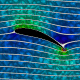Here is an animation that shows how the streamlines change when you increase the circulation around the airfoil. (Please note: The background fluid motion in this animation is just for effect and is not accurate!) Here is some Mathematica code to plot the streamlines and pressure using Bernoulli’s equation:
```(* runtime: 13 seconds *) U = rho = 1; chord = 4; thk = 0.5; alpha = Pi/9; y0 = 0.2; x0 = -thk/5.2; L = chord/4; a = Sqrt[y0^2 + L^2]; gamma = 4Pi a U Sin[alpha + ArcCos[L/a]]; w[z_, sign_] := Module[{zeta = (z + sign Sqrt[z^2 - 4 L^2])/2}, zeta = (zeta - x0 - I y0)Exp[-I alpha]/Sqrt[(1 - x0)^2 + y0^2]; U(zeta + a^2/zeta) + I gamma Log[zeta]/(2Pi)]; sign[z_] := Sign[Re[z]]If[Abs[Re[z]] < chord/2 && 0 < Im[z] < 2y0(1 - (2Re[z]/chord)^2), -1, 1];w[z_] := w[z, sign[z]]; V[z_] = D[w[z, sign], z] /. sign -> sign[z]; << Graphics`Master`; DisplayTogether[DensityPlot[-0.5rho Abs[V[(x + I y)Exp[I alpha]]]^2, {x, -3, 3}, {y, -3, 3}, PlotPoints -> 275, Mesh -> False, Frame -> False, ColorFunction -> (If[# == 1, Hue[0, 0, 0], Hue[(5# - 1)/6]] &)],ContourPlot[Im[w[(x + I y)Exp[I alpha]]], {x, -3, 3}, {y, -3, 3}, Contours -> Table[x^3 + 0.0208, {x, -2, 2, 0.1}], PlotPoints -> 100, ContourShading -> False], AspectRatio -> Automatic];```

Advertisements
29
Oct
07

### Zoom AnimationGoogle Maps is lots of fun.

29
Jun
07

### Fundamental Modes of Vibration for a Sunset Moth-shaped membrane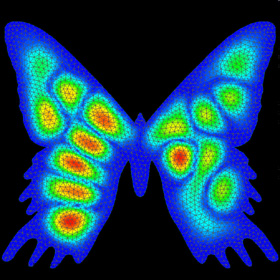Mode displacement plot of the 18th mode of a Sunset Moth-Shaped Membrane. NEiWorks. Just for fun.
06
Jun
07

### FEM Solution to Poisson’s equation on Quad Mesh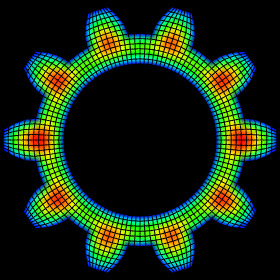FEM Solution to Poisson’s equation on Quad Mesh – Mathematica 4.2, AutoCAD 2000, 6/10/07
Here is some code to solve Poisson’s equation on an unstructured grid of quadrilateral elements:
```(* runtime: 0.05 second *) n = 10; nodes = Flatten[Table[{x, y}, {x, -1, 1,2.0/(n - 1)}, {y, -1, 1, 2.0/(n - 1)}], 1]; nnode = Length[nodes]; elements =Flatten[Table[{i, i + 1, i + n + 1, i + n} + (j - 1) n, {j, 1, n - 1}, {i, 1, n - 1}], 1]; nelem = Length[elements]; fixed = Map[Position[nodes, #][[1, 1]] &, Select[nodes, Max[Abs[#]] == 1 &]]; Kglobal = Table[0, {nnode}, {nnode}]; F = phi = Table[0, {nnode}]; Scan[(plist = nodes[[#]]; dphi = {plist[] - plist[],plist[] - plist[]}; B = Inverse[Transpose[dphi].dphi]; C1 = {{2, -2}, {-2, 2}}B[[1, 1]] + {{3, 0}, {0, -3}}B[[1, 2]] + {{2, 1}, {1,2}}B[[2, 2]];C2 = {{-1, 1}, {1, -1}}B[[1, 1]] + {{-3, 0}, {0, 3}} B[[1, 2]] + {{-1, -2}, {-2, -1}}B[[2, 2]]; Kglobal[[#, #]] += Det[dphi]Join[Thread[Join[C1, C2]], Thread[Join[C2, C1]]]/6; F[[#]] += Det[Join[{{1, 1, 1}}, Transpose[nodes[[#[[{1, 2, 3}]], {1, 2}]]]]]/4) &, elements]; free = Complement[Range[nnode], fixed]; phi[[free]] = LinearSolve[Kglobal[[free, free]], F[[free]]]; Mean[x_] := Plus @@ x/Length[x]; PlotColor[x_] := Hue[2(1 - x)/3]; Show[Graphics[Table[{PlotColor[Mean[phi[[elements[[i]]]]]/Max[phi]], Polygon[nodes[[elements[[i]]]]]}, {i, 1, nelem}], AspectRatio -> 1]];```

Links

02
Mar
07

### CFM56-5 Turbofan Jet Engine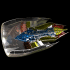I saw this cool looking poster of a turbofan engine schematic and it inspired me to make a 3D rendering of it. This engine is used for the Airbus. The streamlines shown here are just for visual effect. In reality, the streamlines would be turbulent. The blue streamlines show where cold air enters and bypasses the engine (turbofans typically have a high bypass ratio). Green streamlines are shown through the compressor stages. The red streamlines show where hot gas exits the burners and turbine stage.CFM56-5 Turbofan Jet Engine – drawn in AutoCAD 2000, AutoLisp, rendered in POV-Ray 3.6.1, 3/2/07

01
Mar
07

### Turbofan BladesThis was rendered from a turbofan blade model that was studied in our CFD lab.

01
Feb
07

### Supersonic FlowHere are some weird tests of CFL3D. The color represents the Mach number.Supersonic Flow – CFL3D (Computational Fluids Laboratory 3D), Tecplot 360

## Welcome !

You will find here some of my favorite hobbies and interests, especially science and art.

I hope you enjoy it!

Subscribe to theRSS feed to stay informed when I publish something new here.

I would love to hear from you! Please feel free to send me an email : bugman123-at-gmail-dot-com

## Recent CommentsBerna Blalack on Magnetic Pendulum Strange…Daan on Magnetic Pendulum Strange…Sebastian Schepis on Diffusion Limited Aggregation…mohammad_andito on CFM56-5 Turbofan Jet Engi…SasQ on Magnetic Field of a Solen… OUPblog » Blog… on Diamond Light Dispersion Complex Roots on Polynomial Roots Joukowski airfoils |… on Joukowski AirfoilKarim Alame on Flapping WingREJISH J on Joukowski Airfoil SOLINOID | Materials… on Magnetic Field of a Solen…Emanuele on 4D “Squarry” Julia…Emanuele on Hydrogen Electron Orbital Prob…Tim on Mandelbrot Set Pickover S…khankasi1 on 4D Quaternion Mandelbrot …

• 561,072 hits
Advertisements